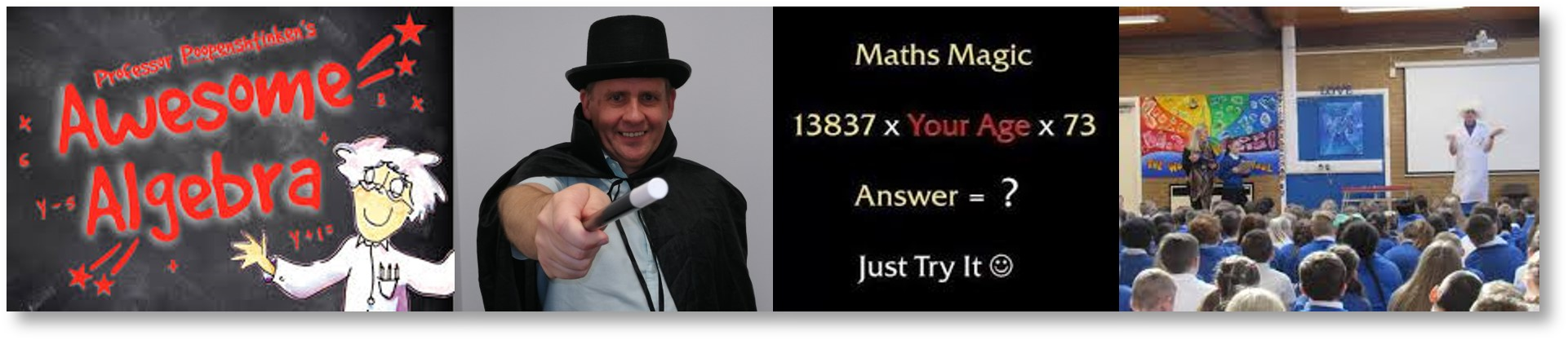# Magical Maths South Manchester# Making mathematics magical for primary school children

### Click here to download a free key stage 1 & 2 video lesson from Roderick Benson or watch the videos on this webpage below.

Free key stage 1 lesson video: Learning tricks to add 2 single digit numbers quickly in your head.

This video is suitable for older key stage 1 children (2nd grade) or younger key stage 2 children. The aim of this video is to teach children mathematical shortcuts to calculating the sum of two single digit numbers. For example, adding 9 to a number is the same as adding 10 to a number and subtracting one. This video also explores all the possible answers to all the possible sums you can make when adding two single digit numbers together. The sums that are easily calculated are like stepping stones in a river where the children then can get to the harder sums by adding or taking away either a 1 or a 2 from the easy to calculated sums. Another example of this would be the sum of 6+7. We know that 6+6 is twelve and so therefore 6+7 must be 13 as 7 is one more than 6.

Free key stage 2 lesson video: First Steps in Algebra.

This video is suitable for key stage 2 students who are about to begin learning algebra. The video introduces the children to the concept that whatever you do to one side of an equation you must also do to the other side of the equation in order to keep the equation balanced. An obvious analogy here, which I use in this video, is a pair of weighing scales where I show how if you add a twenty penny piece to one side, then you must also add a twenty penny piece to the other side in order for the scales to remain balanced. Teaching the children this principle helps them to understand exactly how algebra works by first principles so that the children not only learn a technique for solving algebraic equations, but also understand exactly why the technique actually works.

Show Contact Form:

## Information Videos about Magical Maths for Teachers

### Key Stage 2 Optional Gifted at Maths Club

Key stage 1 virtual club activity video made during Covid-19 lockdown

Key stage 2 virtual club activity video made during Covid-19 lockdown

Safe Guarding, Insurance and other Company Information